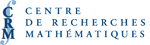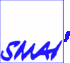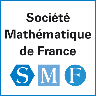# PARTENAIRESNouveaux logiciels pour la solution numérique d'équations différentielles (SCMAI)
Org: Paul Muir (St. Mary's) et Ray Spiteri (Saskatchewan)
[PDF]

TONY HUMPHRIES, McGill University, Montreal
COLFDE: A differential-difference equation BVP Solver
[PDF]

We describe a fortran collocation boundary value problem solver in the COLSYS family of codes, for state-dependent delay and advanced-delay differential equation BVPs. Advanced-delay problems arise in a number of applications, including as the defining equations for travelling waves on a lattice. At first sight, since we solve all the equations in one go, advanced terms create no more difficulty in coding a BVP solver than do delayed terms, and the presence of both will just create off diagonal terms in the Jacobian matrix for the Newton iteration. However, advanced-delay equations are ill-posed as initial value problems, and the existence of solutions of BVPs is delicate, and so the discretization needs to be handled carefully. We will describe a number of issues that arise including the need for and implementation of implicit boundary functions, the breakdown of the sparsity structure in the numerical linear algebra, small Newton convergence balls, and problems associated with state-dependency of solutions. Computations of lattice differential equations, a Neuronal network and a Bando traffic model will illustrate the issues.

PAUL MUIR, Saint Mary's University, Halifax, Nova Scotia, Canada
New Software for the Numerical Solution of Differential Equations
[PDF]

The first part of this talk will provide an overview of the topics to be discussed by the speakers in this minisymposium. The talks will consider software packages for the numerical solution of differential equations, including ones for the interval computation of the solution of DAE systems, the numerical solution of delay differential equations, and the numerical solution of boundary value ODEs in several computing environments such as Matlab, C++, and Fortran.

The second part of the talk will focus on one such project involving a recent developed Fortran 95 package, called BVP__SOLVER, for the numerical solution of boundary value ODEs, written by the author together with Larry Shampine. This package uses defect control as the primary means of controlling the quality of the numerical solution but also provides an option for estimation of the global error as a post-processing step. BVP__SOLVER makes uses of a number of features of the Fortran 95 language to enhance the user-friendliness of the code.

An overview of the capabilities of the new package will be followed by a brief description of recent work focused on improving the defect estimation algorithm employed by BVP__SOLVER. The code along with a number of sample application drivers is available at http://cs.smu.ca/~muir/BVP_SOLVER_Webpage.shtml.

NED NEDIALKOV, McMaster University, Hamilton, Canada
DAETS: a Differential-Algebraic Equation code in C++ for high index and high accuracy
[PDF]

Ned Nedialkov and John Pryce (Cranfield University, UK) are the authors of DAETS, a C++ code for solving differential-algebraic equations (DAEs). It uses Pryce's structural analysis theory, and expands the solution in Taylor series using automatic differentiation. DAETS is very effective when high accuracy is required, and at solving problems of high index-we have solved artificial DAEs of index up to 47. It is versatile: higher-order systems do not have to be cast in first-order form; it can solve explicit and implicit ODEs; it can solve purely algebraic problems, by simple or by arc-length continuation.

This talk will outline the theory and algorithms behind DAETs and the code structure of DAETS. We give examples of code's performance on standard test problems, an index-15 DAE, and a pure algebraic system solved as an implicit index-1 DAE using continuation.

Towards automatically generated variational integrators
[PDF]

Many fundamental physical systems have variational formulations, such as mechanical systems in their Lagrangian formulation. Direct discretization of the relevant variational principles leads to what are called variational integrators. For many systems this generates (implicit) symplectic and momentum preserving one step integration methods. However, such methods can be very complicated and time consuming to implement.

I will describe some advances in the basic theory of variational integrators, which allow us to automatically convert any ordinary one step method into a variational integrator of the same order. I will also describe some work in progress towards a system which does that.

HOSSEIN ZIVARI PIRAN, University of Toronto, Toronto, Ontario
Developing a User-Friendly Delay Differential Equations Modeling Package
[PDF]

Ideally a delay differential equations (DDEs) modeling package should provide facilities for approximating the solution, performing a sensitivity analysis and estimating unknown parameters. We propose new techniques for efficient simulation, accurate sensitivity analysis and reliable parameter estimation of DDEs. Using these techniques, we have implemented a new software package DDEM. The package is programmed in C++ and intended to provide user-friendly calling sequences. The preliminary numerical results are very satisfying and show the effectiveness of the techniques.

GREG REID, University of Western Ontario
Geometric Algorithms for PDAE and DAE
[PDF]

Highly nonlinear implicit DAE and PDAE occur frequently in applications. Algorithms that treat such systems almost always make two key assumptions:

The system is (i.e., number equations = number unknown functions)
There is effectively only 1 irreducible component (or manifold) of solutions

Any algorithm that claims completeness, must be able to determine solutions of systems with more than 1 component and also deal with non-square systems. I will describe progress on designing such algorithms, and also related progress on implementation. I will start with the algebraic case, and numerically stable methods from the new area of numerical algebraic geometry, based on rigorous globally convergent homotopy continuation methods. Then I will discuss the extension of these continuation methods and their implementation to systems of DAE and PDAE. The flavour will be introductory and the geometric ideas illustrated visually, and with examples.

This is joint work with my collaborator Wenyuan Wu.

LARRY SHAMPINE, Southern Methodist University, Dallas, TX 75275
BVP Solver that Controls Residual and Error
[PDF]

Programs for the numerical solution of boundary value problems (BVPs) for ordinary differential equations generally try to control the true error. BVP solvers require users to provide guesses for the desired solution and a mesh that reveals its behavior. Because these guesses may be poor, the asymptotic approximations that justify error estimates may not be very good, or perhaps not even valid. This is of particular concern when a user asks for only modest accuracy. A more robust approach is to control a residual in the numerical solution because it is possible to estimate it reliably, no matter how bad the guesses. This is the approach taken in bvp4c, the MATLAB BVP solver. Unfortunately control of the residual controls only indirectly the true error that is of most interest to users. Nevertheless, we show that for some methods, a control of a kind of residual controls the true error directly. Our investigation has resulted in a new BVP solver called bvp5c. It is used exactly like bvp4c and has some advantages in addition to a robust and more natural control of error.

WARREN WECKESSER
VFGEN: A Code Generation Tool for Vector Fields
[PDF]

VFGEN is a program that generates computer code for ordinary and delay differential equations. From a single definition file of the user's equations, VFGEN can generate code for: IVP solver libraries such as CVODE, LSODA and DDE_SOLVER; numerical continuation and bifurcation analysis programs such as AUTO, MATCONT and PDDE-CONT; and general purpose computing environments such as MATLAB, Octave and Scilab. VFGEN automates the tedious and error-prone task of implementing the vector field in computer code. Symbolic differentiation is used to generate Jacobians and higher derivatives. For many of the output formats, VFGEN can also generate a working demonstration program.

In this talk, I will give an overview of VFGEN. Demonstrations will show how VFGEN makes it easy to use a wide variety of computational tools in the numerical study of a system of ordinary or delay differential equations.